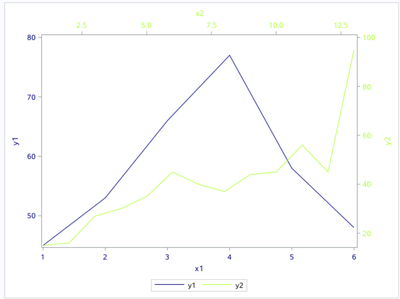## PROC SGPLOT with two x axis

I have two different datasets and want to create a plot with two line graph. The X and Y axis in these two datasets are different. Is it possible to add two X and two Y axis simultaneously in one graph. I found its possible to add two Y axis in PROC SGPLOT. Is there any way to do the same for two X axis using SGPLOT or other SAS procedures?

data have;
input
x1 y1;
datalines;
1 45
2 53
3 66
4 77
5 58
6 48
;
data have2;
input
x2 y2;
datalines;
1 15
2 16
3 27
4 30
5 35
6 45
7 40
8 37
9 44
10 45
11 56
12 45
13 95
;

proc sgplot data=have;
series x=x1 y=y1;
run;

proc sgplot data=have2;
series x=x2 y=y2;
run;

1 ACCEPTED SOLUTION

Accepted SolutionsKsharp
Super User

## Re: PROC SGPLOT with two x axis

``````data have1;
input
x1 y1;
datalines;
1 45
2 53
3 66
4 77
5 58
6 48
;
data have2;
input x2 y2;
datalines;
1 15
2 16
3 27
4 30
5 35
6 45
7 40
8 37
9 44
10 45
11 56
12 45
13 95
;

data want;
merge have1 have2;
run;

proc sgplot data=want;
series x=x1 y=y1/lineattrs=(color=navy);
series x=x2 y=y2/x2axis y2axis lineattrs=(color=greenyellow);
xaxis valueattrs=(color=navy) labelattrs=(color=navy);
yaxis valueattrs=(color=navy) labelattrs=(color=navy);
x2axis valueattrs=(color=greenyellow) labelattrs=(color=greenyellow);
y2axis valueattrs=(color=greenyellow) labelattrs=(color=greenyellow);
run;``````4 REPLIES 4

## Re: PROC SGPLOT with two x axis

First would be to combine the data sets.

Depending upon the final desired appearance there a MANY options but this is a basic graph with both lines:

```data toplot;
set have  (in=in1)
have2 (in=in2)
;
run;

proc sgplot data=toplot;
series x=x1 y=y1;
series x=x2 y=y2;
run;```

Labels, axis controls, style settings for lines/markers might help make the result more usable.

## Re: PROC SGPLOT with two x axis

Thank you!Ksharp
Super User

## Re: PROC SGPLOT with two x axis

``````data have1;
input
x1 y1;
datalines;
1 45
2 53
3 66
4 77
5 58
6 48
;
data have2;
input x2 y2;
datalines;
1 15
2 16
3 27
4 30
5 35
6 45
7 40
8 37
9 44
10 45
11 56
12 45
13 95
;

data want;
merge have1 have2;
run;

proc sgplot data=want;
series x=x1 y=y1/lineattrs=(color=navy);
series x=x2 y=y2/x2axis y2axis lineattrs=(color=greenyellow);
xaxis valueattrs=(color=navy) labelattrs=(color=navy);
yaxis valueattrs=(color=navy) labelattrs=(color=navy);
x2axis valueattrs=(color=greenyellow) labelattrs=(color=greenyellow);
y2axis valueattrs=(color=greenyellow) labelattrs=(color=greenyellow);
run;``````## Re: PROC SGPLOT with two x axis

Thank you! It creates exactly what I was looking for.
Discussion stats
• 4 replies
• 1900 views
• 1 like
• 3 in conversation